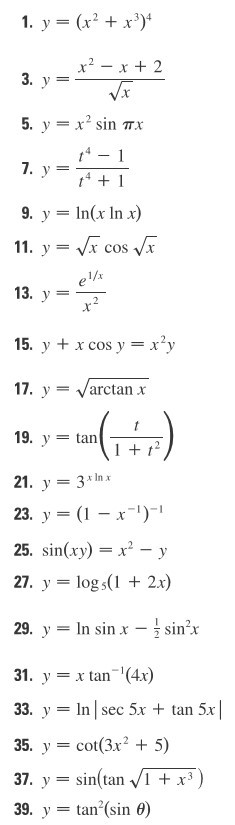# 1. У (x2 + x3)4 9. У ln(x ln x) 1 + t 21. У 3x1n х 23, y = (1-x-1)- 25. sin(xy)-x2-y 27. y-logs 2x) 29. y--In sin x--sin-х 1. yan (4x) 33. yInsec 5x + tan 5x 35, y = cot(3x2 + 5) 37. y=sin(tan-/I +x3

Question

Differentiation#29help_outlineImage Transcriptionclose1. У (x2 + x3)4 9. У ln(x ln x) 1 + t 21. У 3x1n х 23, y = (1-x-1)- 25. sin(xy)-x2-y 27. y-logs 2x) 29. y--In sin x--sin-х 1. yan (4x) 33. yInsec 5x + tan 5x 35, y = cot(3x2 + 5) 37. y=sin(tan-/I +x3 fullscreen

### Want to see the step-by-step answer?